## Example Questions

1 3 Next →

### Example Question #21 : How To Find The Length Of A Line With Distance Formula

Find the length of a line segment whose endpoints are located at the coordinates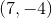and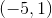.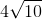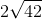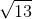Explanation:

According to the distance formula, the linear distancebetween two points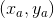and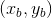is given by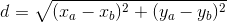,

which is a generalization of the Pythagorean Theorem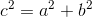for any two points on the coordinate plane.

The two points given in this problem areand. Although the question asks for the length of a line segment given its two endpoints, the distance formula is applicable here because the geometric meaning of "distance between two points" is the length of a line segment. Hence, we can use the distance formula to determine the distance between these two endpoints as shown: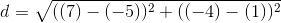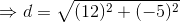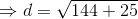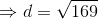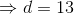Hence, the line segment bounded by the pointsandisunits long.

### Example Question #22 : How To Find The Length Of A Line With Distance Formula

One line has four collinear points in order from left to right A, B, C, D.  If AB = 10’, CD was twice as long as AB, and AC = 25’, how long is AD?

40'

50'

45'

30'

35'

45'

Explanation:

AB = 10 ’

BC = AC – AB = 25’ – 10’ = 15’

CD = 2 * AB = 2 * 10’ = 20 ’

AD = AB + BC + CD = 10’ + 15’ + 20’ = 45’

1 3 Next →